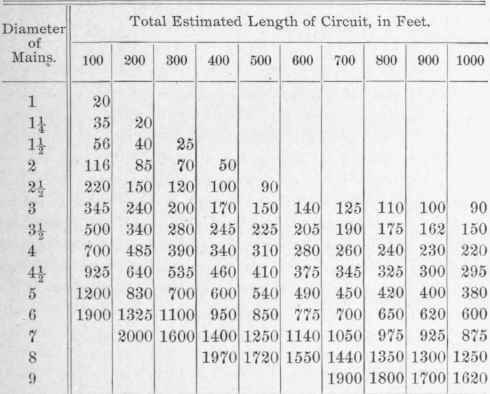213. There are two methods of determining the proper sizes of hot-water piping, as follows: In the first method, the amount of heat to be emitted from the radiators per minute is ascertained and divided by 166.7, which gives the number of gallons of water required per minute with a fall of temperature of 20°. If any other range of temperature is adopted, this divisor must be changed to suit. Having found the flow required, the diameter of the pipes is then computed by the ordinary laws of hydraulics.

214. The second method is by the use of tables, which are prepared from data furnished by experience in actual practice, rather than from the data derived from theoretical investigation. It is assumed that each radiator will always operate at its maximum capacity, and that the fall in temperature of the water will be 20°. Therefore, the table is based upon the area of the radiator surfaces, instead of upon the heat units emitted.

The first method is by far the more accurate, but it requires considerable computation, and in practice it is unnecessary that the pipes should be so accurately sized.

The second method is convenient, and is better adapted to the various compound-circuit systems. The error, if any, is always in favor of a freer circulation of the water, so that the fall of temperature will be somewhat less than 20°, and the radiators will then emit a little more heat than rated.

## 215. Mains

Mains. The following table shows the amount of heating surface that can be properly supplied with hot water by mains of a given size and uniform diameter throughout their whole length, the radiators being located upon the first floor. The fall of temperature is assumed to be 20°, and the height of the circuit is between 10 and 15 feet. The amount of radiator surface that can be maintained on higher floors may be found by multiplying the amount shown in the table by the factors given in Art. 210.

## Table 18. Size Of Hot-Water Mains## 216. Risers

Risers. Table 19 shows the area of radiator surface, in square feet, that can be properly supplied at various elevations by risers of a given diameter. The radiators are supposed to be connected by ordinary short connections having a total length of about 10 feet. Each story corresponds to a height of about 10 feet. Fall of temperature, 20°.

## Table 19. Size Of Hot-Water Risers## 217. Size Of Radiator Connections

Size Of Radiator Connections. In the following table the area of radiator surface is given, which is adapted to connections having the diameter given, for service on the first floor, that is, at an elevation between 10 and 15 feet above the level of the return connection to the boiler. It is assumed that the aggregate length of the flow and return connections is about 10 feet, and also that they include six elbows, or their equivalents, the resistance offered by such connections to the flow of water being the same as that of 100 feet of plain, straight pipe. Fall of temperature, 20°.